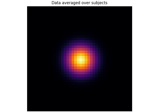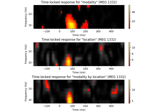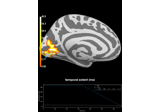# mne.stats.f_threshold_mway_rm#

mne.stats.f_threshold_mway_rm(n_subjects, factor_levels, effects='A*B', pvalue=0.05)[source]#

Compute F-value thresholds for a two-way ANOVA.

Parameters:
n_subjects`int`

The number of subjects to be analyzed.

factor_levelslist-like

The number of levels per factor.

effects`str`

A string denoting the effect to be returned. The following mapping is currently supported:

• `'A'`: main effect of A

• `'B'`: main effect of B

• `'A:B'`: interaction effect

• `'A+B'`: both main effects

• `'A*B'`: all three effects

pvalue`float`

The p-value to be thresholded.

Returns:
F_threshold

List of F-values for each effect if the number of effects requested > 2, else float.

Notes

New in version 0.10.

## Examples using `mne.stats.f_threshold_mway_rm`#Statistical inference

Statistical inferenceMass-univariate twoway repeated measures ANOVA on single trial power

Mass-univariate twoway repeated measures ANOVA on single trial powerRepeated measures ANOVA on source data with spatio-temporal clustering

Repeated measures ANOVA on source data with spatio-temporal clustering